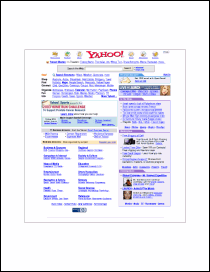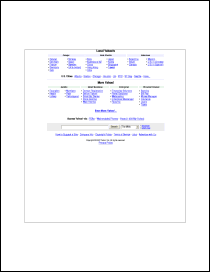AddImageToChain FunctionAdds a new page from a paged HTML or PostScript render.Syntax

 [C#] `int AddImageToChain(int id)` [Visual Basic] `Function AddImageToChain(id As Integer) As Integer` ```may throw Exception()```Params

 Name Description id The ID of the previous object in the chain. return The ID of the newly added object.NotesExample

This example shows how to import an HTML page into a multi-page PDF document.

We first create a Doc object and inset the edges a little so that the HTML will appear in the middle of the page.

[C#]
```Doc theDoc = new Doc(); theDoc.Rect.Inset(72, 144);```
[Visual Basic]
```Dim theDoc As Doc = New Doc() theDoc.Rect.Inset(72, 144)```

We add the first page of HTML. We save the returned ID as this will be used to add subsequent pages.

[C#]
```int theID; theID = theDoc.AddImageUrl("http://www.yahoo.com/");```
[Visual Basic]
```Dim theID As Integer theID = theDoc.AddImageUrl("http://www.yahoo.com/")```

We now chain subsequent pages together. We stop when we reach a page which wasn't truncated.

[C#]
```while (true) {   theDoc.FrameRect();   if (!theDoc.Chainable(theID))     break;   theDoc.Page = theDoc.AddPage();   theID = theDoc.AddImageToChain(theID); }```
[Visual Basic]
```While True   theDoc.FrameRect()   If Not theDoc.Chainable(theID) Then     Exit While   End If   theDoc.Page = theDoc.AddPage()   theID = theDoc.AddImageToChain(theID) End While```

After adding the pages we can flatten them. We can't do this until after the pages have been added because flattening will invalidate our previous ID and break the chain.

[C#]
```for (int i = 1; i <= theDoc.PageCount; i++) {   theDoc.PageNumber = i;   theDoc.Flatten(); }```
[Visual Basic]
```For i As Integer = 1 To theDoc.PageCount   theDoc.PageNumber = i   theDoc.Flatten() Next```

Finally we save.

[C#]``` theDoc.Save(Server.MapPath("pagedhtml.pdf")); theDoc.Clear();```
[Visual Basic]
```theDoc.Save(Server.MapPath("pagedhtml.pdf")) theDoc.Clear()```

We get the following output.pagedhtml.pdf [Page 1]pagedhtml.pdf [Page 2]